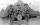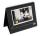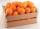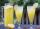Sweets

Mom bought box of sweets for their children.Whole package of 100 sweets divided among 4 their children so that each child receives the most and for she remains the least sweets. How many sweets left for mom.

Result

x =  0

Solution:Leave us a comment of this math problem and its solution (i.e. if it is still somewhat unclear...):Be the first to comment!Next similar examples:

1. Trio56 children lined up in groups of three. How many children did not create a trio?
2. Date gameLet now is Wednesday. What day is after 248 days? Write the result as a number: 1=Monday, 2=Tuesday, 3=Wednesday, 4=Thursday, 5=Friday, 6=Saturday, 7=Sunday?
3. Math classificationIn 3A class are 27 students. One-third got a B in math and the rest got A. How many students received a B in math?
4. Product of two fractionsProduct of two fractions is 9 3/5 . If one of the fraction is 9 3/7. Find the other fraction.
5. The resultHow many times I decrease the number 1632 to get the result 24?
6. Crates 2One crate will hold 50 oranges. If Bob needs to ship 932 oranges, how many crates will he need?
7. Fractions 4How many 2/3s are in 6?
8. Pizza 5You have 2/4 of a pizza and you want to share it equally between 2 people how much pizza does each person get?
9. DivisionWhich number in division 16 give 12 and the rest 3?How many 1/4 cup servings are in 2 and 1/3 cups of lemonade?
11. Double 5Peter was thinking of a number. Peter doubles it and gets an answer of 8.6. What was the original number?
12. LineCan we construct a line segment, if we know: center
13. ZdeněkZdeněk picked up 15 l of water from a 100-liter full-water barrel. Write a fraction of what part of Zdeněk's water he picked.
14. ExpressionSolve for a specified variable: P=a+4b+3c, for a
15. Forest nurseryIn the forest nursery after winter, they found that 1/10 stems died out of them. For them, they land 193 new spruces. How many spruces are in the forest nursery?
16. In fractionsAn ant climbs 2/5 of the pole on the first hour and climbs 1/4 of the pole on the next hour. What part of the pole does the ant climb in two hours?
17. Addition of Roman numbersAdded together and write as decimal number: LXVII + MLXIV